# How Newton's Laws Relate to the Law of Conservation of Energy & Momentum

Lesson Transcript
Instructor: Damien Howard

Damien has a master's degree in physics and has taught physics lab to college students.

The many different topics under physics can be connected in ways you might not expect. Here you'll learn how Newton's second and third laws help us to derive conservation of energy and momentum. Updated: 07/08/2019

## Connecting Physics Topics

For teaching purposes, physics is split into different categories. There are the main topics like classical mechanics, thermodynamics, electricity and magnetism, and quantum mechanics. Even these large topics are broken down further into smaller ones. For example, in classical mechanics, you'll learn about linear motion, rotational motion, forces, energy, and momentum all as subtopics.

It's easy to think of these subtopics as completely separate concepts that have nothing to do with each other, but this isn't true. Physics teaches us how the natural world works around us, and all of these concepts combine to form that understanding.

These topics are often connected in ways we might not even realize. Let's see how Newton's laws of motion relate to two examples, conservation of energy and conservation of momentum.

An error occurred trying to load this video.

Try refreshing the page, or contact customer support.

Coming up next: Pressure: Definition, Units, and Conversions

### You're on a roll. Keep up the good work!

Replay
Your next lesson will play in 10 seconds
• 0:04 Connecting Physics Topics
• 0:55 Conservation of Energy
• 3:35 Conservation of Momentum
• 5:12 Lesson Summary
Save Save

Want to watch this again later?

Log in or sign up to add this lesson to a Custom Course.

Timeline
Autoplay
Autoplay
Speed Speed

## Conservation of Energy

For conservation of energy, we're going to focus on Newton's second law, which tells us that the acceleration of an object is directly proportional to the net force acting on it, and indirectly proportional to its mass.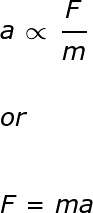This law can be used to help show that the law of conservation of energy is true. To do this, let's start with reviewing what exactly that law entails.

The law of conservation of energy states that the total energy in an isolated system remains constant over time. Mathematically, we can write this out as the total energy at time two (E2) minus the total energy at time one (E1) divided by the change in time between the two (Δt) equals zero.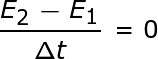Now, remember that total energy equals kinetic energy (KE) plus potential energy (PE).We've rearranged the equation so that the left-hand side consists of two separate parts, one for KE and one for PE. In order to show that the conservation of energy is true, we're going to show that the left-hand side does in fact equal zero. Let's start by looking at only the KE part of the left-hand side.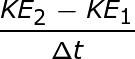Recall that KE = (1/2)mv2 where m is mass and v is velocity.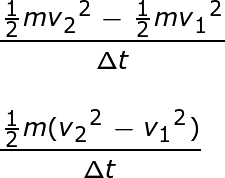With some algebra we can find that (v22 - v12) = (v2 - v1)(v2 + v1).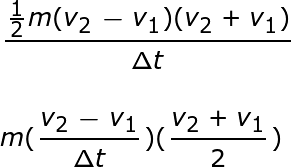Here (v2 + v1)/2 is average velocity (v), and (v2-v1) / Δt is a change in velocity over time, which is acceleration (a).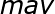This is where Newton's second law finally comes into play. It tells us that F = ma, so the kinetic energy portion of our equation can be written as: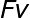Now, let's move onto the potential energy portion of the equation.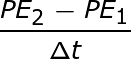PE2 - PE1 is a change in potential energy (ΔPE). A change in potential energy is related to work (W) and force through two formulas: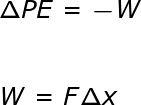Combining these two formulas with the potential energy portion of the equation results in: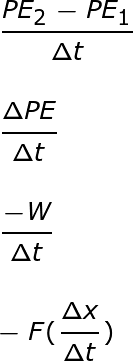A change in position (Δx) divided by a change in time (Δt) is equal to average velocity.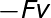Finally, we take what we found and insert it back into our original formula.We found that the left-hand side of the equation equals the right-hand side. With the help of Newton's 2nd law, we've shown that the conservation of energy holds true.

To unlock this lesson you must be a Study.com Member.
Create your account

### Register to view this lesson

Are you a student or a teacher?

### Unlock Your Education

#### See for yourself why 30 million people use Study.com

##### Become a Study.com member and start learning now.
Back
What teachers are saying about Study.com
Create an account to start this course today
Used by over 30 million students worldwide